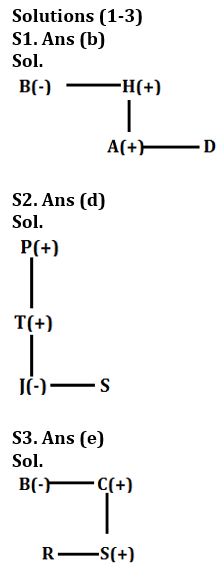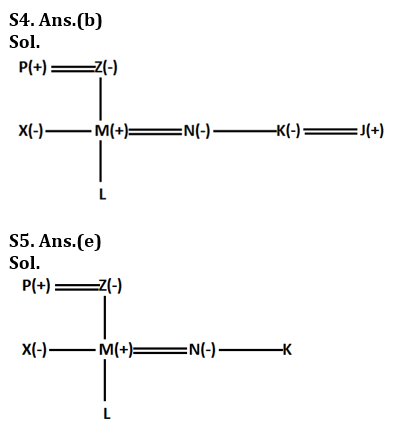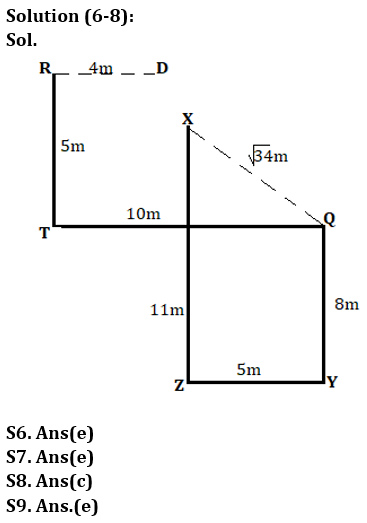# IDBI AM/ Bank of India PO Reasoning Quiz 2023 -19 March

Topic – Practice Set

Directions,(1-3) Study the following information carefully and answer the questions given below.

‘P – Q’ means ‘P is the father of Q’.
‘P÷ Q’ means ‘P is sister of Q’.
‘P × Q’ means ‘P is the mother of Q’.
‘P + Q’ means ‘P is brother of Q’.

Q1. Which of the following means ‘AB is nephew’?
(a) A + C – B × K
(b) B÷ H – A + D
(c) B ÷ G – A ÷ R
(d) B + T × A ÷ E
(e) None of these

Q2. Which of the following means ‘P is grandfather of J’?
(a) J÷ W – U – P
(b) P × G + J÷ A
(c) P – B ÷ J ÷ R
(d) P – T – J÷S
(e) None of these

Q3. How is R related to B in the expression ‘B÷C – S + R’?
(a) aunt
(b) Nice
(c) Nephew
(d) None of these
(e) Cannot be determined

Directions (4-5): Study the information carefully and answer the questions given below.
P is father of M who is brother-in-law of K. N is the daughter-in-law of Z, who is the grandmother of L. P has only two children one boy and one girl. X is sister-in-law of N. P is father-in-law of N. K is single.
Q4. If K is the wife of J, what is the relationship of J with respect to N?
(A brother
(b) Brother-in-law
(c) Uncle
(d) Cannot be determined
(e) None of these

Q5. What is the relationship of L with respect to X?
(a) Nephew
(b) Nice
(c) Son
(d) Daughter
(e) Cannot be determined

Directions (6-8): Study the information carefully and answer the questions given below.

Ravi starts traveling from point R and walks 5m towards south and reaches point T. He takes a left turn and walks 10 m to reach point Q. From point Q, he takes a right turn and walks 8m to reach point Y. From point Y, he takes a right turn and walks 5m to reach point Z. From point Z, he starts walking towards north and walks 11m to reach point X.

Q6. In which direction is point Y from point T?
(a) North West
(b) Ans
(c) South West
(d) South
(e) None of these

Q7. What is the shortest distance between X and Q?
(a) 5 m
(b) 6 m
(c) 4 m
(d) 3 m
(e) None of these
Q8. If point D is 4m east of point R, what is the direction of point D with respect to point Z?
(a) Ans
(b) North East
(c) North West
(d) South
(e) None of these

Q9. Given statement T≥C>H≥QWhich of the following is definitely true if A is definitely true?
(a) A≤U
(b) C ≥ Q
(c) H > Y
(d) Y=W
(e) None is true

Q10. If each letter in the word ‘CONFERENCE’ is arranged alphabetically from left to right, how many letters will remain unchanged?
(a) One
(b) Five
(c) Two
(d) Three
(e) Four

Q11. Gopal is 20th from the left end of a row and Geetansh is 25th from the right end of the row. If they interchange their positions, Geetansha will be 14th from the right end. Find the total number of persons in the queue?
(a) 32
(b) 35
(c) 34
(d) 33
(e) None of these

Directions (12-14): Study the following information carefully and answer the given questions.

Each of the six ropes A, B, C, D, E and F are of different lengths. Height of D is higher than E and lower than B. The height of A is higher than C and lower than B. The height of F is greater than that of B. The height of C is greater than D. The length of the second longest rope is 16 m and the length of the third shortest rope is 9 m. Height of D is 7 m.

Q12. Which of the following is the third longest rope?
(ac
(b) B
(c)
(d) d
(e) Cannot be determined

Q13. What can be the sum of the lengths of E and B?
(a) 23
(b) 24
(c) 21
(d) 25
(e) None of these

Q14. How many cords are longer than F?
(a) One
(b) Two
(c) Three
(d) None
(e) Cannot be determined

Q15. If ‘white black yellow’ is coded as ‘mk bl po’ and ‘orange black white’ is coded as ‘mk pogn’, then which of the following is the code for ‘black’?
(a) mk
(b) g
(c) Po
(d) None of these
(e) Either (a) or (c)

Solution:Sol.Original word- Council

Sol.Total number of persons in the row = (20+14-1) = 33

Solutions (12-14):
Sol. The arrangement will be: F>B(16m)>A>C(9m)>D(7m)>E
S12.Ans(c)
S13.Ans(c)
S14.Ans(d)